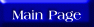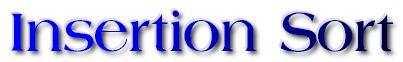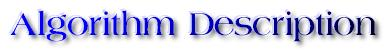One of the simplest sorting algorithms is the insertion sort. The code for the algorithm is as follows:

for(i = 1; i < n; i++){
Inserted = false; //boolean variable
j = i;
while((j >= 1) && (Inserted == false){
if(List[j] < List[j-1])
swap(List[j], List[j-1]);
else
Inserted=true;
j--;
}
}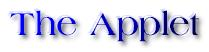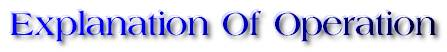This algorithm works by first considering the first element of the list (element 0) to be correctly placed. It proceeds to the next element (element 1) and compares its value with. If the magnitude is smaller, it swaps it with element 0. Now, elements 0 and 1 are sorted, but only with respect to each other. Element 2 is now considered, and is moved to its correct position with respect to elements 0 and 1, i.e., it may stay where it currently is, or it may be placed between what are now elements 0 and 1, or it may be placed before element 0. To do this, elements 2 and 1 may be swapped, resulting in the placement of element 2 between what is currently element 0 and element 1, and then another swap between what are now elements 0 and 1 may be done to place the element before what is now element 0. This continues over and over again, considering the elements from element 3 through the last element of the list. Each element is placed in its proper position in the part of the list already consider to be sorted. Each element under consideration is swapped with its immediately preceeding neighbour as it moves towards the front of the list. The moment the swapping stops, the element's immediately preceding neighbor has lesser magnitude, and so the current element may be considered to be properly placed.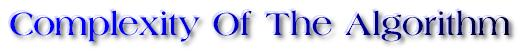In this method there are N-1 insert operations. The j-th insert operation inserts a new element into a sorted array of j elements and hence takes at most j comparisions. Thus the total number of comparisions are:

1 + 2 + 3 + ... + (N-1) = N*(N-1)/2 = O(N^2)